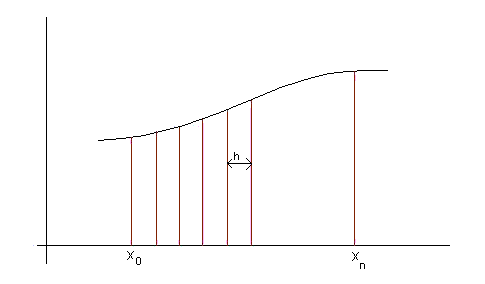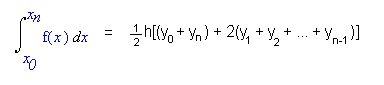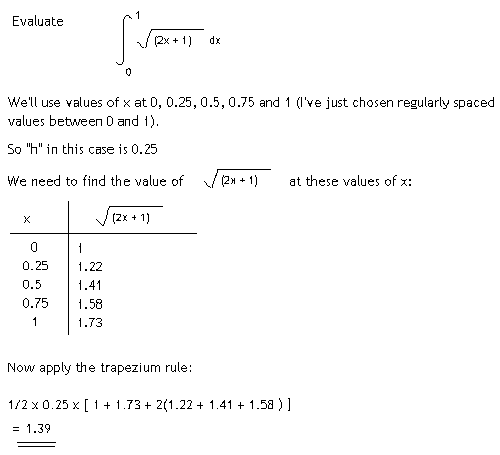## The Trapezium Rule

The trapezium rule is a way of estimating the area under a curve. We know that the area under a curve is given by integration, so the trapezium rule gives a method of estimating integrals. This is useful when we come across integrals that we don't know how to evaluate.

The trapezium rule works by splitting the area under a curve into a number of trapeziums, which we know the area of.If we want to find the area under a curve between the points x0 and xn, we divide this interval up into smaller intervals, each of which has length h (see diagram above).

Then we find that:where y0 = f(x0) and y1 = f(x1) etc

If the original interval was split up into n smaller intervals, then h is given by: h = (xn - x0)/n

Example